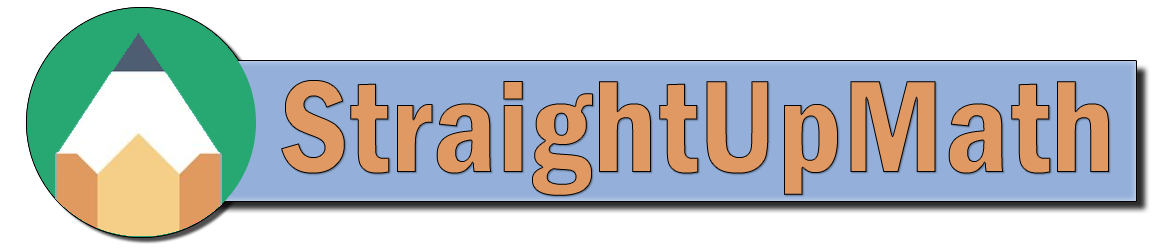Multiplying Decimals(L1)

Sorry, no video for this problem at this time.

## Practice

Find the product.
a)    9.92 × 0.3 =
2.976

b)    6.56 × 7 =
45.92

c)    3.45 × 0.9 =
3.105

d)    8.6 × 0.3 =
2.58

e)    7.93 × 3 =
23.79

f)    5.56 × 6 =
33.36

g)    2 × 0.2 =
0.4

h)    3.56 × 0.8 =
2.848

i)    5.35 × 0.6 =
3.21

j)    6.91 × 0.4 =
2.764